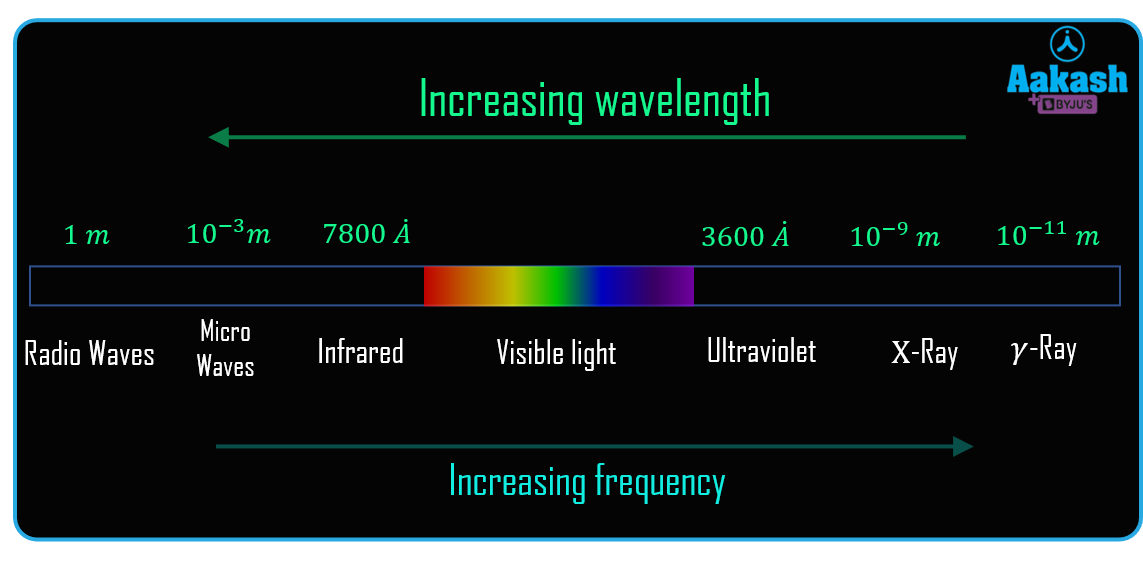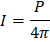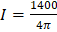• Call Now

1800-102-2727•

# Unit of light

What if you turn on the bulb of your room and tell them to give you 1 kg of light. Is it a correct statement? The answer is obviously no. The light is not a physical quantity like mass, weight, length etc. So we can not measure the light directly. We can measure the intensity of light. There are many units in which the intensity of light can be measured. Let's see them !

Table of content

• Light
• Unit and measurement of light
• Lumen (lm)
• Candela (cd)
• Lux (lx)
• Practice problem
• FAQs

## Light

Light or visible light is electromagnetic radiation that is the part of the electromagnetic spectrum. The electromagnetic spectrum consists of radiation from the -rays to the radio waves. As we can see from the name, electromagnetic radiation is the oscillation of electric and magnetic fields. Electric field oscillation and magnetic field oscillation are perpendicular to each other and also perpendicular to the direction of wave propagation. Light is just another kind of energy like -rays and radio waves.

Visible light is the only radiation that is visible. It helps living beings such as humans and animals to see things around. The natural sunlight called white light, consists of all the visible radiation. We can see all the radiation in the form of different colours when white light passes through a prism. The light normally travels in a straight line but it can reflect and refract and change the direction accordingly when it comes to the context of some obstacle.The visible light has the wavelength from 3600 Å to 7800 Å or from 360 nm to 780 nm. In the increasing order of the wavelength the visible colours of the light are violet, indigo, blue, green, yellow, orange and red. The frequency of visible light is from 430-750 terahertz.

Light can also be considered a particle or packet of energy called a photon. The photon is the massless particle that travels with wavelike properties. The photon is the smallest quantity of energy and measure of quanta of light. This concept where we deal with the both wave and particle nature of the light is called the wave-particle duality of light and is the origin of quantum mechanics.

## Unit and measurement of light

While talking about the unit of the light, it seems meaningless as light is not a physical quantity and it cannot be measured. Although the light consists of various physical quantities such as wavelength, frequency, speed, intensity etc. that can be measured. So the unit of light is the units of these physical quantities.

The light is mainly measured in two different sets of units : 1. Radiometry and 2. Photometry

1. Radiometry : In radiometry the light is measured in terms of power or intensity for all the wavelengths.
2. Photometry : In photometry the light is measured for those wavelengths which are useful for human use.

There are three main units of light which are given below,

1. Candela
2. Lumen
3. Lux

Let's discuss all three one by one.

## Lumen (lm)

The lumen is the unit to measure the luminous energy per unit time or luminous power emitted from the source of light, which is also known as luminous flux. It is denoted by the symbol (lm). Luminous flux differs from the radiant flux. The Luminous flux consists of the wavelength as per the model of sensitivity of the human eye, called luminosity function while the radiant flux consists of the radiation of all the wavelengths.

## Candela (cd)

Candela is the fundamental unit of luminous intensity accepted by the international system of units (SI).The candela is luminous power per unit solid angle emitted by the point source in a specific direction. It is represented by the group of small letters (cd). It is equal to (lm/sr). Hence Cendela is the quantification of luminous power per unit solid angle discharged from the source in a specific direction. The candela is not measured in luminous intensity by considering all the wavelengths of the spectrum but with the wavelength as per the model of sensitivity of the human eye, called luminosity function. One candela is given by the luminous intensity of light emitted from the standard wax candle. A frequency of approximately 540 x 1012 Hz corresponding wavelength 555 nm, is used to define the candela because the human eye is most sensitive around this particular frequency. The candela is expressed by taking the value of luminous intensity 683 expressed in the unit (lm W-1) , that is equal to cd kg-1 m-2 s3.
1 cd = 1 lm/sr.

## Lux (lx)

A lux is the unit to measure the illuminance or luminous existence of light; it is given as energy per unit time per unit area or luminous flux per unit area emitted from the source. It is equal to 1 lumen per metre square. Like the other unit of luminous light it is also defined as per the model of sensitivity of the human eye, called luminosity function. It measures the intensity of light that hits a surface of consideration.
1 lx = 1 lm/m2 = 1 cd sr /m2

## Practice Problems

Q1. A bulb 50 W gives the light of 1400 Lumens. If the light is equally radiant in all directions, find the luminous intensity in candela.

A. Given luminous power P=1400 lm

As the light is radiant in all direction so the solid angle will be equal to the 4π

The luminous intensity of lightI=111.41 cd

Hence the luminous intensity is 111.41 cd.

Q2. The luminous intensity of a light source is 150 cd What is the luminous power of the sources?

A. Given I = 150 cd

The solid angle is 4π

The luminous power of the source $P=4\pi ×I$

Hence the luminous power of the source is 1885 lm.

Q3. A source of light is placed in the space limiting a flux of 1000 lm. If it is light up the area of 5 m2 then find the illuminance of light.

A. Given luminous flux or luminous power P = 1000 lm

And Area A = 5 m2

The illuminance of the light $E=\frac{P}{A}=\frac{1000}{5}$

E = 200 lx

Hence the illuminance of the light source is 200 lx

Q4. An area of 2.27 m2 is lighted by the a light of source of illuminance 317 lx. What is the total flux of the light source?

A. Given Area A=2.27 m2
Illuminance E=317 lx

The total flux of the light source is                     ϕ=E×A

Hence the total flux through light source is 719.59 lm.

## FAQs

Q1. What are the various units to measure the light intensity?
A.
The intensity of light is measured in candela, lumens, lux etc.

Q2. How much is one candela?
A.
A source is said to emit one candela if its intensity matches with the intensity with the standard candle. In other words when the luminous power of 1 lm is incident in a direction then the luminous intensity associated with the 1 steradian solid angle is equal to 1 candela.

Q3. According to quantum theory what is a light?
A.
According to quantum theory light is a bundle of packets of energy called photons which move with a high speed.

Q4. The colour of the visible light depends on which quantity?
A.
The colour of visible light can be specified by the wavelength and frequency of the wave. Visible light has the seven colours as violet, indigo, blue, green, yellow, orange and red.

The wavelength of varies from 4000 Å of violet colour to 7000 Å of red colour. The frequency varies from 750 THz of violet colour to 429 THz of red colour.Talk to our expert
Resend OTP Timer =
By submitting up, I agree to receive all the Whatsapp communication on my registered number and Aakash terms and conditions and privacy policy